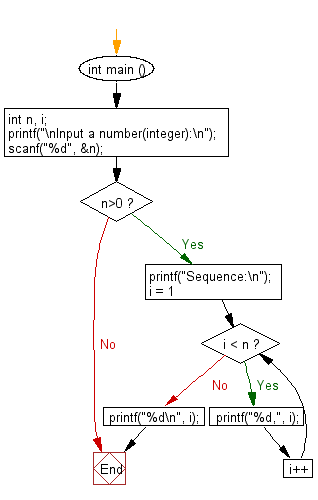﻿ C : Add a comma between two numbers, no comma after the last

# C Exercises: Insert a comma between two numbers, no comma after the last character

## C Basic Declarations and Expressions: Exercise-120 with Solution

Write a C program to print a sequence from 1 to a given (integer) number, inserting a comma between these numbers. There will be no comma after the last character.

Sample Solution:

C Code:

``````#include <stdio.h>

int main () {
int n, i;

// Prompt user for input
printf("\nInput a number(integer):\n");

// Read an integer value 'n' from user
scanf("%d", &n);

// Check if 'n' is positive
if (n > 0) {

printf("Sequence:\n");

// Loop to print numbers from 1 to 'n-1'
for (i = 1; i < n; i++) {
printf("%d,", i); // Print each number followed by a comma
}

printf("%d\n", i); // Print the last number without a comma
}
}
``````

Sample Output:

```Input a number(integer):
25
Sequence:
1,2,3,4,5,6,7,8,9,10,11,12,13,14,15,16,17,18,19,20,21,22,23,24,25
```

Flowchart:C programming Code Editor:

What is the difficulty level of this exercise?

Test your Programming skills with w3resource's quiz.

﻿